• +91 9971497814
• info@interviewmaterial.com

# RD Chapter 15- Areas Related to Circles Ex-15.2 Interview Questions Answers

### Related Subjects

Question 1 : Find, in terms of π the length of the arc that subtends an angle of 30° at the centre of a circle of radius 4 cm.

Angle at the centre subtended an arc = 30°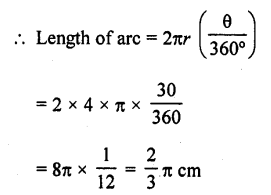Question 2 : Find the angle subtended at the centre of a circle of radius 5 cm by an arc of length (5π/3 cm.

Radius of the circle (r) = 5 cm 571
Length of arc = 5π/3 cm
Let θ be the angle subtended by the arc, then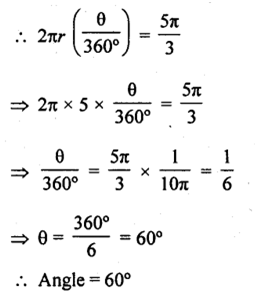Question 3 : An arc of length 20tc cm subtends an angle of 144° at the centre of a circle. Find the radius of the circle.

Length of an arc = 20π cm
Angle subtended by the arc = 144°
Let r be the radius of the circle, then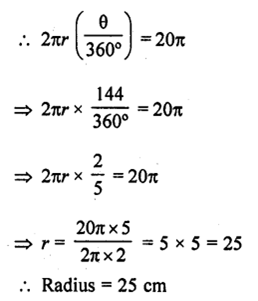Question 4 : An arc of length 15 cm subtends an angle of 45° at the centre of a circle. Find in terms of π ; the radius of the circle.

Length of arc = 15 cm
Angle subtended at the centre = 45°
Let r be the radius of the circle, then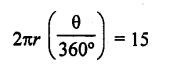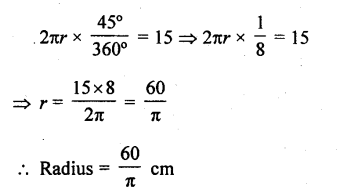Question 5 : Find the angle subtended at the centre of a circle of radius ‘a’  by an arc of length (aπ/4)  cm.

Radius of the circle (r) = a cm
Length of arc = aπ/4   cm
Let θ be the angle subtended by the arc at the centre, then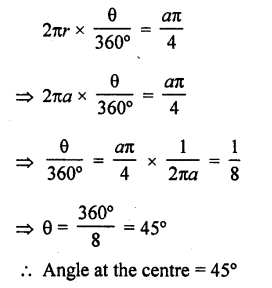Question 6 : A sector of a circle of radius 4 cm contains an angle of 30°. Find the area of the sector.

Radius of the sector of a circle (r) = 4 cm
Angle at the centre (θ) = 30°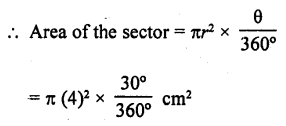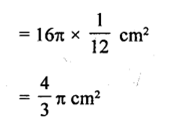Question 7 : A sector of a circle of radius 8 cm contains an angle of 135°. Find the area of the sector.

Radius of the sector of the circle (r) = 8 cm
Angle at the centre (θ) = 135°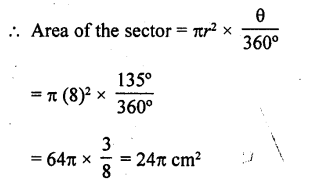Question 8 : The area of a sector of a circle of radius 2 cm is 7 is cm2. Find the angle contained by the sector.

Area of the sector of a circle =π cm2
Radius of the circle (r) = 2 cm
Let 0 be the angle at the centre, then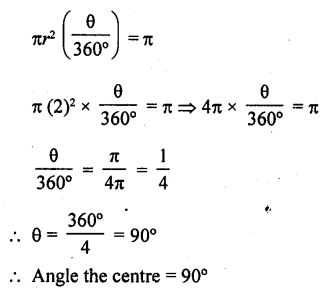Question 9 : The area of a sector of a circle of radius 5 cm is 5π cm2. Find the angle contained by the sector.

Area of the sector of a circle = 5π cm2
Radius of the circle (r) = 5 cm
Let 9 be the angle at the centre, then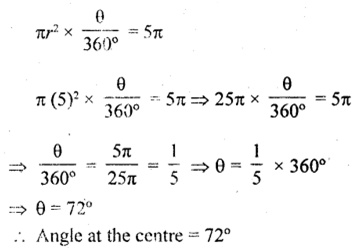Question 10 : Find the area of the sector of a circle of radius 5 cm, if the corresponding arc length is 3.5 cm. [NCERT Exemplar]

Let the central angle of the sector be θ.
Given that, radius of the sector of a circle (r) = 5 cm
and arc length (l) = 3.5 cm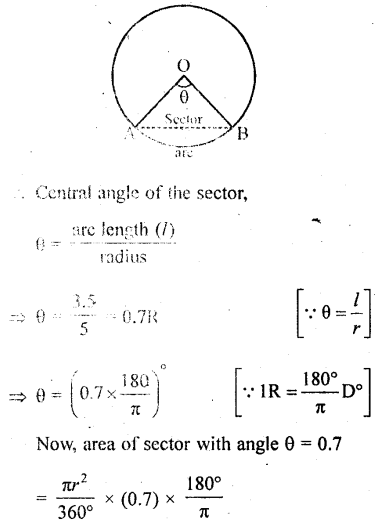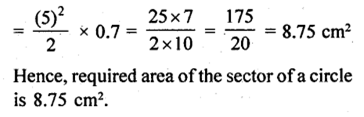Todays Deals### RD Chapter 15- Areas Related to Circles Ex-15.2 Contributorskrishan

Name:
Email:

# Latest News# 9000 interview questions in different categories